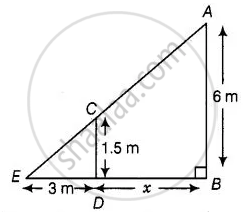# A street light bulb is fixed on a pole 6 m above the level of the street. If a woman of height 1.5 m casts a shadow of 3 m, find how far she is away from the base of the pole. - Mathematics

Sum

A street light bulb is fixed on a pole 6 m above the level of the street. If a woman of height 1.5 m casts a shadow of 3 m, find how far she is away from the base of the pole.

#### Solution

Let A be the position of the street bulb fixed on a pole AB = 6 m and CD = 1.5 m be the height of a woman and her shadow be ED = 3 m.

Let distance between pole and woman be x m.Here, woman and pole both are standing vertically.

So, CD || AB

In ΔCDE and ΔABE,

∠E = ∠E   ......[Common angle]

∠ABE = ∠CDE   ......[Each equal to 90°]

∴ ΔCDE ∼ ΔABE   .....[Bt AAA similarity criterion]

Then, (ED)/(EB) = (CD)/(AB)

⇒ 3/(3 + x) = 15/6

⇒ 3 xx 6 = 1.5(3 + x)

⇒ 18 = 1.5 xx 3 + 1.5x

⇒ 15=.5x = 18 - 4.5

∴ x = (13.5)/1.5 = 9 m

Hence, she is at the distance of 9 m from the base of the pole.

Concept: Similarity of Triangles
Is there an error in this question or solution?

#### APPEARS IN

NCERT Mathematics Exemplar Class 10
Chapter 6 Triangles
Exercise 6.4 | Q 8 | Page 74
Share